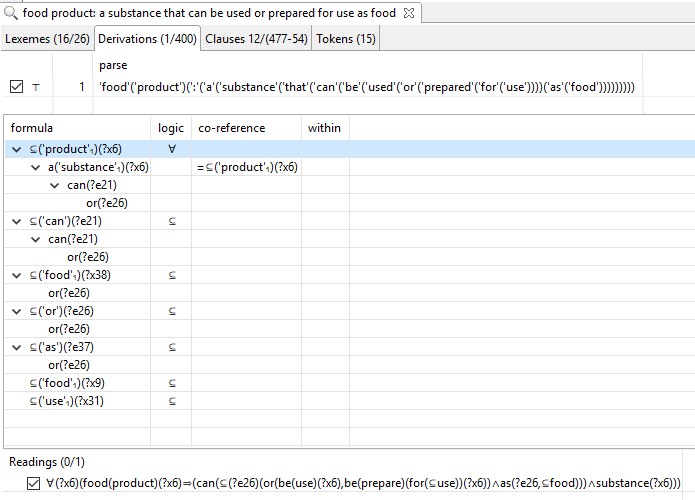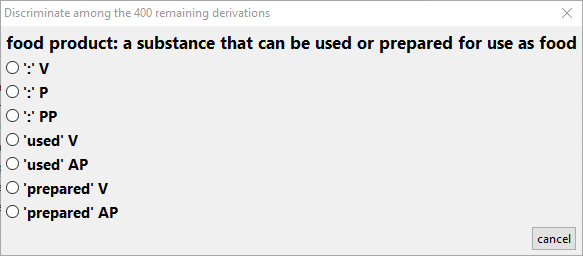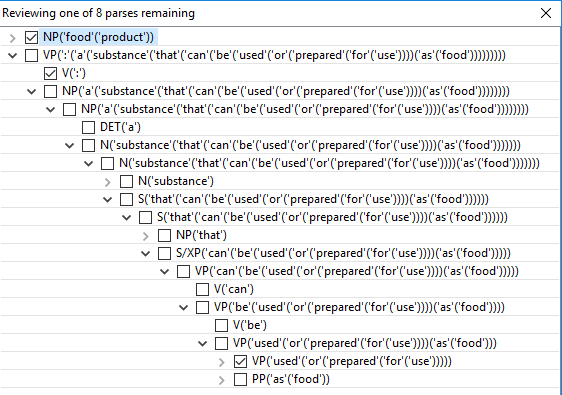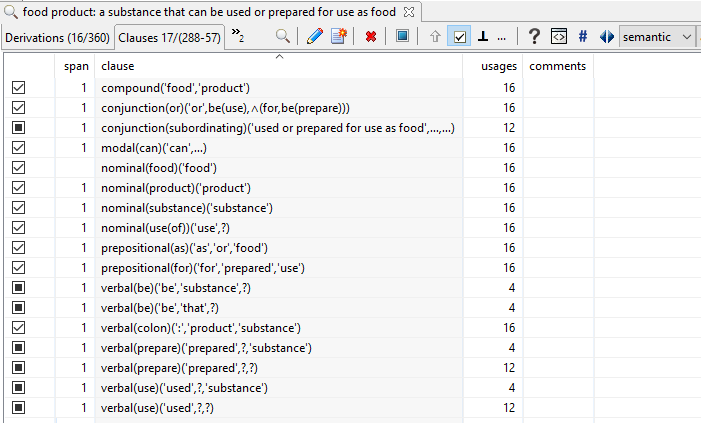# Dictionary Knowledge Acquisition

The following is motivated by Section 6359 of the California Sales and Use Tax.  It demonstrates how knowledge can be acquired from dictionary definitions:Here, we’ve taken a definition from WordNet and prefixed it with the word followed by a colon and parsed it using the Linguist.

The lexical and syntactic ambiguities of this sentence are significant.  On the first parse, over 400 derivations are returned.  If the software is configured to clarify parts of speech, it then presents the following dialog:And after a couple of clicks, we help the parser understand the ‘or’ by clicking on the verb phrase shown below:As you can see, this leaves only 8 parses.  Just to be safe, we reparse using the constraints we’ve provided and find less than 400 parses, meaning that we can be more confident that the correct parse is among those we review.

With parsing configured in one of its more permissible modes, there are still 16 parses with the correct parse tree structure.  The following clauses allow us to discriminate among those semantically:Users are encouraged not to worry about why these exist but to click on those that they find easiest to affirm or reject.  So, skimming down the list very quickly:

• affirm the verbal for ‘used’ or ‘prepare” with ‘substance’ as its second argument
• i.e., the direct object of either is the substance
• note: the subject of either is implicit and unspecified in this sentence

Either affirmation eliminates the remaining semantic ambiguity and leaves 4 derivations which are equivalent except for linguistic details that are rarely of interest to most users (in which case users simply select the best derivation).

Finally, the quantification on the defined term (i.e., before the colon) becomes universal and the subject and object of the colon (interpreted as in the verb ‘is’ or ‘means’) are unified to produce the logic shown first above.

• ∀(?x6)(food(product)(?x6)⇒(can(⊆(?e26)(or(be(use)(?x6),be(prepare)(for(⊆use))(?x6))∧as(?e26,⊆food)))∧substance(?x6)))

In this rendering, certain predicates are “conflated” and various details (e.g., elided variables and unreferenced situations) are omitted.  This makes the logic much easier to read and easier to comprehend.  If you want to know even more, read on, but the following is more than most are interested in.

Here is the same logic rendered without conflation:

• ∀(?x6)(⊆(?x9)(food(?x9)(compound(?x6,?x9)∧product(?x6)))⇒(can(⊆(?x38)(food(?x38)⊆(?e26)(or(be(use)(?x6),⊆(?x31)(use(?x31)⊆(?e29)(for(?e29,?x31)∧be(prepare)(?e29)(?x6))))∧as(?e26,?x38))))∧substance(?x6)))

And here is the same logic when elided arguments and unreferenced situations are rendered:

• ∀(?x6)(⊆(?x9)(food(?x9)(compound(?x6,?x9)∧product(?x6)))⇒(can(⊆(?x38)(food(?x38)⊆(?e26)(or(⊆(?i24)be(use)(?i24,?x6),⊆(?x31)(⊆(?i36)use(of)(?x31,?i36)⊆(?e29)⊆(?i28)(for(?e29,?x31)∧be(prepare)(?e29)(?i28,?x6))))∧as(?e26,?x38))))∧substance(?x6)))

In this logical rendering of the knowledge, ‘⊆’ is an under-specified quantifier.  For the logically inclined, it’s semantics is not necessarily limited to existential or universal quantification, as in first-order predicate calculus.

• For example, this literal within the formula may be interpreted as referring to food in general (i.e., as a type) rather than a first-order individual:  as(?e26,⊆food)

Note that ‘e’ variables denote what linguists call ‘events’ (see Parsons 90) but which are more easily understood as ‘situations’.  (Non-linguists tend to differ with linguists on whether states or processes are ‘events’ but less so that they are ‘situations’.)

• In ⊆(?e26)(or(be(use)(?x6),be(prepare)(for(⊆use))(?x6))∧as(?e26,⊆food)), ?e26 denotes the disjunction itself (not either of its disjuncts), which is complement by the ‘as’.

This is in keeping with Hobbs’ “Ontological Promiscuity” and demonstrates that Davidsonian variables are not limited to what we may intuitively consider an ‘event’.